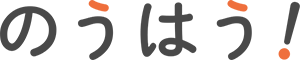# Units commonly used in personal computersThe unit used when representing the performance of your PC and Internet speed.
Do not select somehow larger numbers? However, as a large number of proportional to the price rise. Let us know the unit properly you need to?

b Bit (bit). The smallest of the binary value. 0 And represented 1.

In the B and b when Byte and bit notation.
B Byte (Byte ). 1 Byte = 8 bits. They can represent the numbers from 0 to 255, if characters, space characters.

１文字 width is 2 bytes.
KB ( kiloByte )-kilobytes.

1 KB = 1024 bytes.
MB -MB (MegaByte).

1 MB = 1024 kilobytes.
GB ( GigaByte ) / GB.

1 Gigabyte = 1024 megabytes.
TB ( TeraByte ) and terabytes.

1 TB = 1024 GB.
BPI ( bits per inch )-bits per inch.

The number of bit per 1 inch.
BPP ( bits per pixel )-bits per pixel. The number of bits per 1 pixel.

image can express pretty, capacity would be larger than bpp,.
bps -Bits / second, ビーピーエス ( bits per second ).

The number of bits can be transferred in 1 seconds.
DPI -Dots / inch, deeper is ( dots per inch ). The number of dots per 1 inch. A unit that represents the image and print output resolution.

Image that appears over larger dots.
FPS -Frames / second, エフピーエス ( frames per second ). The number of frames per second of video or video.

Higher smooth motion can be expressed.
Hz ( Hertz )-Hertz.

International unit of frequency.
Point -Point. 1 / 72 Inch.

Font size unit.
RPM And all PEEM rounds / minute. Rotating disk or fan unit for 1 minute of rotation unit. Mainly used in the representation of hard disk performance.